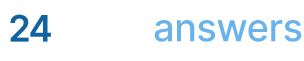# Algebraic Geometry

## Homework Help & Tutoring

We offer an array of different online Algebraic Geometry tutors, all of whom are advanced in their fields and highly qualified to instruct you.Send your subject help request Submit your homework problem, or a general tutoring request.
Get quotes from qualified tutors Receive a response from one of our tutors as soon as possible, sometimes within minutes!
Algebraic Geometry Tutors Available Now
55 tutors available
Algebraic Geometry Homework Library
8 total solutions
See what our students are saying
Can you help me with my homework in less than 24 hours?
Can you help me with my exam/quiz/test?
How much will it cost?
What kind of payments do you accept?

# Algebraic Geometry

Algebraic Geometry

Algebraic geometry is the study of the geometric properties of solutions to polynomial equations including solutions in dimensions beyond three. This branch of mathematics evolved from analytical geometry after 1850 when topology, complex analysis, and algebra were used to study algebraic curves. An algebraic curve is the graph of an equation f (x, y) =0 with f (x, y) considered a polynomial in two complex variables and cannot be factored. Curves are classified by a non-negative integer known as the genus (g) that cannot be calculated from their polynomial.

In the 20th century, it was discovered that basic ideas of classical algebraic geometry could be applied to what are known as commutative rings with the algebra being the ring of polynomials and the geometry derived from the set of zeroes of polynomials called an algebraic variety. Commutative rings have units such as integers and the geometry of a commutative ring is determined by its algebraic structure: in particular its prime ideals. The mathematician Grothendieck defined schemes as these basic geometric objects which have the same relationship to the geometry of a ring as a manifold (a topological space that is Euclidean) to a coordinate chart (a way of expressing the points of a small neighborhood usually on a manifold).

At around the same period, the language of category theory emerged. Category theory is the branch of mathematics that formalizes a number of algebraic properties of collections of changes within mathematical objects such as binary relations, groups, sets, and topological spaces. This branch emerged from the increasingly abstract nature of algebraic geometry.

Some theorems that exist within algebraic geometry include Bezout’s theorem which states that two curves (C and D in a polynomial) are defined by equations of degrees M and N intersecting at MN points. In essence this theorem states that every two lines intersect at a unique point. Another theorem is the Riemann-Roch theorem which uses integrals along paths on C (algebraic curve) to characterize g (genus) analytically. Grothendieck extended the Riemann-Roch theorem in the 1950s by generalizing varieties (the solution set of r polynomial equations in n complex variables) to schemes.

Some disciplines within algebraic geometry include birational geometry, curves, algebraic groups, Abelian varieties, arithmetic algebraic geometry, and computational aspects. Birational geometry involves analysis of the isomorphic (corresponding or similar in form and relationship) class of an object. Algebraic groups discuss the symmetric groups of real objects and are essentially matrix group schemes and allow the various tools of algebraic geometry to be applied to their study. Abelian varieties help to generalize elliptic curves with such curves having led to advances within many fields of mathematics. Arithmetic algebraic geometry is the study of arithmetical problems using algebraic geometry. Computational aspects involve calculations with schemes in algebra.

Algebraic geometry can be applied to the field of integer programming or used in statistics within game theory, geometric modeling, matching graphs, and matching codes for error correction. The most significant application of algebraic geometry is with geometry as algebraic equations used instead of making exact graphs.

Sources

1. https://www.britannica.com/science/algebraic-geometry; accessed July 20 and 21
2. http://mathworld.wolfram.com/AlgebraicGeometry.html; accessed July 20 and 21
3. http://mathworld.wolfram.com/CategoryTheory.html; accessed July 20
4. http://mathworld.wolfram.com/Manifold.html; accessed July 20
5. http://mathworld.wolfram.com/CoordinateChart.html; accessed July 20
6. http://www.math.mcgill.ca/goren/AGwebpage/webpage2.htm; accessed July 21
7. https://web.archive.org/web/20040415021548/http://planetmath.org/encyclopedia/AlgebraicGeometry.html; accessed July 21
8. http://www.mathcaptain.com/geometry/algebraic-geometry.html; accessed July 23

## College Algebraic Geometry Homework Help

Since we have tutors in all Algebraic Geometry related topics, we can provide a range of different services. Our online Algebraic Geometry tutors will:

• Provide specific insight for homework assignments.
• Review broad conceptual ideas and chapters.
• Simplify complex topics into digestible pieces of information.
• Answer any Algebraic Geometry related questions.
• Tailor instruction to fit your style of learning.

With these capabilities, our college Algebraic Geometry tutors will give you the tools you need to gain a comprehensive knowledge of Algebraic Geometry you can use in future courses.

## 24HourAnswers Online Algebraic Geometry Tutors

Our tutors are just as dedicated to your success in class as you are, so they are available around the clock to assist you with questions, homework, exam preparation and any Algebraic Geometry related assignments you need extra help completing.

In addition to gaining access to highly qualified tutors, you'll also strengthen your confidence level in the classroom when you work with us. This newfound confidence will allow you to apply your Algebraic Geometry knowledge in future courses and keep your education progressing smoothly.

Because our college Algebraic Geometry tutors are fully remote, seeking their help is easy. Rather than spend valuable time trying to find a local Algebraic Geometry tutor you can trust, just call on our tutors whenever you need them without any conflicting schedules getting in the way.

Start Working With Our College Algebraic Geometry Tutors
To fulfill our tutoring mission of online education, our college homework help and online tutoring centers are standing by 24/7, ready to assist college students who need homework help with all aspects of Algebraic Geometry.24houranswers.com Parker Paradigms, Inc 5 Penn PLaza, 23rd Floor New York, NY 10001
SUBJECTS COVERED